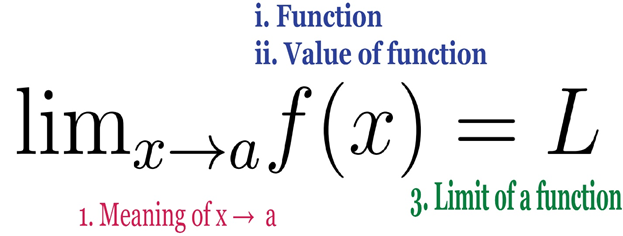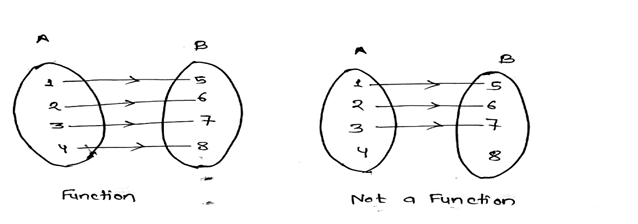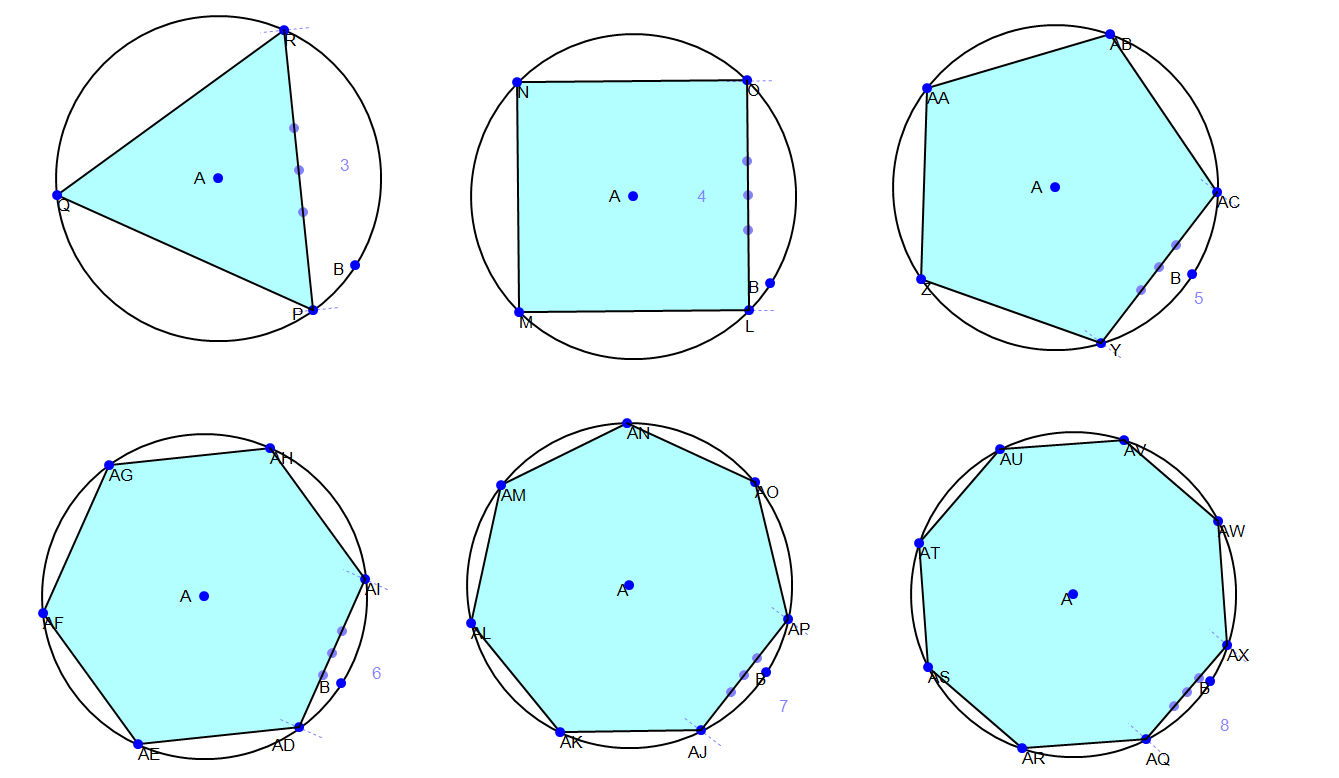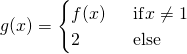# So close, no matter how far: Concept of Limit

3년 전

So close, no matter how far

I’d like to start this post off quoting one of my favorite artists who always inspire me to do maths, Metallica. Yes! I’m not kidding. Distractions can do nothing if you have the power of heavy metal piercing through your soul.

Anyways, you might have probably guessed the theme of this post. If the title did not give you enough clues, here’s a hint. It’s about limits. What exactly is the limit? Something that separates a man from God? Or perhaps you from your superhero? Or maybe you from approaching a girl. Mathematically, limits have the same sense but these senses get quantified into more perceivable forms of what you cannot perceive.

Let me try to get through to you through an anecdote. Think about it like this. A frog tries to get to a well. He’s a lazy from and so, decides to take half the step he previously took. So, will he ever reach the well? Stay tuned to find out! (Now you want to know, don’t you? ) . Now, let's move into real maths.

# Mathematical Explanation

The concept of limit is one of the most important concepts in mathematics. It is the fundamental concept in the development of calculus. Also, it is a simple concept to grasp. I will try to make it even simpler using figures and examples.

Limit, here we mean limit of the function. The mathematical symbol for the limit is given below.(Source: Self-coded in Latex, Edited in illustrator)

It means the limit of the function f(x) at x = a is L.

Some of you might not have an idea of a function. Well, a function is a relation that shows the relation between two different variables. If the time I arrive college depends on the time I wake up, my time of arrival at college is a function of the time I wake up. For a detailed view, let's dive deeper.

# What is the function?

let X and Y be any two non-empty sets. Then the relation from set X to set Y is called the function if every element of set X is associated with a unique element of set Y.

It is denoted byy = f(x),

x is the element of X

y is the element of Y

Let us break down the formal definition;

• Every element of set X should have an association. So, there shouldn’t be any left outs in the set X.

• A unique element of set Y. So, the elements of set X shouldn’t be associated with more than 1 element. There may be an element in set Y which may not be associated with any element.(Source: self=drawn in A4, scanned by camscanner app)

But it’s not only necessary that we know two variables depend on each other. We also need to know HOW they depend on each other. For example, if I wake up a minute earlier, do I reach the college 20 minutes earlier or 30 minutes earlier or even perhaps, an hour later because I’ll be bumping my metallica CD’s again. That is why the value of a function is necessary.

# What is the value of the function?

Let f be the function from set X to set Y and x =a be any element of set X. Then, the value f(a) of set Y in which x=a in set X is associated is called the value of the function f(x) at x=a.

If the value of the function f(a) is finite, then the function exists at x=a.

If the value of function i.e. f(a) is undefined, the function f(x) doesn’t exist or defined for x=a.

Examples:

i. Letbe any function,
then at x=1;is the value of function at x=1;

ii.be any function,

then at x=2;= undefined. So, the function is not defined at x=2.

iii. Letbe any function,

then at x = 1;This is interesting. If any number other than 0 is divided by 0 then it undefined. If any number other than 0 divides 0 then the result is 0. But, here both the numerator and denominator are zero. This doesn’t give any number. This can give any number based on the result that 0n = 0. This form is called an indeterminate form. There are other indeterminate forms too. Eg. http://quicklatex.com/cache3/4b/ql_d4e967c18afd38471105751d6a66554b_l3.png etc.

# Meaning ofLet x be any variable and. x=0.9 is close to 1. But, 0.99 is closer to 1. The closeness increases with the increase in a number of 9s. But, the value will never be 1. That means the numerical difference between 1 and x will be some small non-zero number. We say that the value of x is approaching 1 from left. It is denoted by http://quicklatex.com/cache3/1d/ql_42445de230c43b5dc8a62dd2708f011d_l3.png

Again, let x takes a value of 1.1. It is close to 1. But, 1.01 is closer to 1. The closeness increases with the increase in number if 0 in between numbers. Due to 1 at the end, the value will never be equal to 1. That means, the numerical difference between x and 1 will be some small non-zero number. We say that the value of x is approaching 1 from the right. It is denoted byIn both cases, the value of x is approaching one. We can incorporate both cases if we take an absolute value of the difference of x and 1. In this case, we say that the value of x is approaching 1. It is denoted by.

For the value a, we can write that.

# Examples of limit:

In this section, I will explain famous two examples to give you the intuition of the limit. The first one is using regular polygon inside a circle and other one using a sum of series.

## Using regular polygon inscribed in a circle:

Consider a regular polygon inscribed inside a circle. The first polygon is of course of three side i.e. equilateral triangle. Let us keep the circle constant and increase the value of the number of sides of the polygon. With an increase in the number of sides, the area of polygon increases. But, no matter however large may be the side of the polygon, the area of the polygon will always be less than the area of the circle. We can make the difference between the area of circle and polygon as small as we please by increasing number of sides of the polygon.(Source: Self, Drawn in Math Illustrator)

Let A(n) be the area of the polygon which depends on the number of sides and A be the area of the circle. When n is getting larger, the area A(n) is approaching A. So, we say that limiting value of area A(n) is the area of the circle. It is denoted by;## Using series

Consider the following series;Let S(n) be a sum function which gives the sum of series unto n. Then,Making a table for the other values.

Sum up termsvalue
11
21.5
31.75
41.875
51.9375
61.96875
71.984375
101.998046875
1001.99999999999999999999999999999984222…

From the table, we can see that the sum approaches 2 as we increase the number of terms. However large number we choose the sum will never be 2. We can make the sum sufficiently close to 2 by increasing number of terms. We can prove that the limiting value is 2.It is geometric series with common ration ½. So,S(n) = 2 -When n becomes sufficiently large thenbecomes sufficiently small that we can neglect it. So, the limiting value of the sum of series is 2.

We can denote it by;# Limit of a Function

Consider a function f(x) =Also, consider a sequence of values of x to be 0.5,0.75,0.875,0.9,0.99,0.99,0.999,0.9999 … whose limit is 1.

xf(x)
0.51.5
0.751.75
0.8751.875
0.91.9
0.991.99
0.9991.999
0.99991.9999
0.9999…1.9999…

We can see from the table that the corresponding values of f(x) are 1.5,1.75,1.875,1.9,1.99,1.999,1.9999, .. which goes nearer to 2 when x goes nearer to 1. So, when x is sufficiently close to 1, f(x) is close to 2.

Again, if we consider sequence of values of x to be 1.5,1.25.1.1,1.01,1.001,1.0001… whose limit is 1.

xf(x)
1.52.5
1.252.25
1.12.1
1.012.01
1.0012.001
1.00012.0001
1.000012.00001

We can see from the table that the corresponding values of f(x) are 2.5,2.25, 2.1,2.01,2.001,2.0001, 2.00001 , .. which goes nearer to 2 when x goes nearer to 1. So, when x is sufficiently close to 1, f(x) is close to 2.

that is when x1, f(x) http://quicklatex.com/cache3/a1/ql_87331204d26a4469bb959d23b56235a1_l3.png 2.

i.eHence, we can finally define the limit of the function.

The number ‘l’ to which the value of function f(x) approaches as the x approaches to a certain number ‘a’ is called the limit of the function f(x) at x=a.# Meaning of Infinity

Let us consider a function f(x) =Also, consider a sequence of values of x to be 0.5,0.75,0.875,0.9,0.99,0.99,0.999,0.9999 … whose limit is 1. We can see that corresponding values of f(x) are 2,4, 8, 100, 1000, 1000 ... which is increasing. If we take the value of x nearer to 1, the value of the function will be large enough. We can make the value of x sufficiently closer to 1 such that corresponding value of f(x) will be greater any positive integer. In such case, we can say that when x tends to one, f(x) tends to infinity.# Conclusion

Thus, we see now what a function is and how it can be limited in its sense of value. A limit poses a restriction to a function or even the way the variables in a function, approach a value.

Now that you know about limits, you have known another part of Mathematics you’ll never use in your life ever again? Really?? Hell no! The limit is everywhere. Limits are the very basis of Calculus. “But Bikki, we won’t use calculus ever again either!” Are you for real right now? Calculus is used in all fields from modeling of physical situation to cryptocurrency.

So, Does the frog ever reach the well? I hope to see your answers in the comment.

#References

1. Hass, J., & Weir, M. D. (2008). Thomas' calculus: Early transcendentals. Boston: Pearson Addison-Wesley.

2. Tall, D., & Vinner, S. (1981). Concept image and concept definition in mathematics with particular reference to limits and continuity. Educational studies in mathematics, 12(2), 151-169.

3. Cinvestav, F. H., & Lara-chavez, H. (1999). Limits, continuity and discontinuity of functions from two points of view: That of the teacher and that of the student.

4. Bezuidenhout, J. (2001). Limits and continuity: Some conceptions of first-year students. International journal of mathematical education in science and technology, 32(4), 487-500.

6. http://www.sparknotes.com/math/precalc/continuityandlimits/summary/

7. http://tutorial.math.lamar.edu/Classes/CalcI/Continuity.aspx

Reference mathematical tools

1. Math illustrations

2. www.quicklatex.com

steemstem:

#SteemSTEM is a community driven project which seeks to promote well-written and informative Science, Technology, Engineering and Mathematics posts on Steemit. The project involves curating STEM-related posts through upvoting, resteeming, offering constructive feedback, supporting scientific contests, and other related activities.

DISCORD: https://discord.gg/j29kgjSSort Order:  trending

though i am out of these ideas. it is realy innovative. great job

You could also think about limits as a mathematical object which tells you how to make a function continuous.

For example in your case f(x) =can be extended to the functionObserve that this function is continuous.

In the case when you are dealing with infinity there are mathematical methods which let you apply the same trick.

This is the reason why we consider limits for functions.

·
·  3년 전

First of all, sorry for the late reply
and secondly, thank you for the suggestion.

The suggestion above is related to continuity of the function. I wanted to focus on the just basic concept of limit. Continuity of the function is whole another part. So, if I I ever write about it, I will surely add this.

I think I have properly defined infinity in this post. How to tackle that can be subject of the next post.

·
·

You can substitute continuity in my reply by approaching something. It is not necessary to define it formally.

In terms of a series or sequence your approach makes sense since they are discrete since they are evaluated for 1,2,3,4 etc. But limit in the sense of this function f that you used is in a sense different since you are relying on its continuity by approaching in a continuous sense a certain value.

·
·
·
·  3년 전

The point of using that example was that the limit can exist without function being continuous. we can intuitively see the function is not continuous at x =1, but we are not interested at exactly that point. We are interested in how the values are approaching. I may be relying in the concept of continuity at other points than x=1, but I still dont know why should I make function continuous at x=1.

·
·
·
·

I think you misunderstand my previous comment.

If a function is defined in some interval then the concept of limit is a bit different because you can use different ways to approach the same point. While if it is only discretely defined you can only do this over the integers. So my claim is that it is better to keep it in a discrete setting if you only define the limit in a discrete setting

·  3년 전

Bro professor jastai lekhexau tw khatra post

·
·  3년 전

Thank you so muh for your comment.